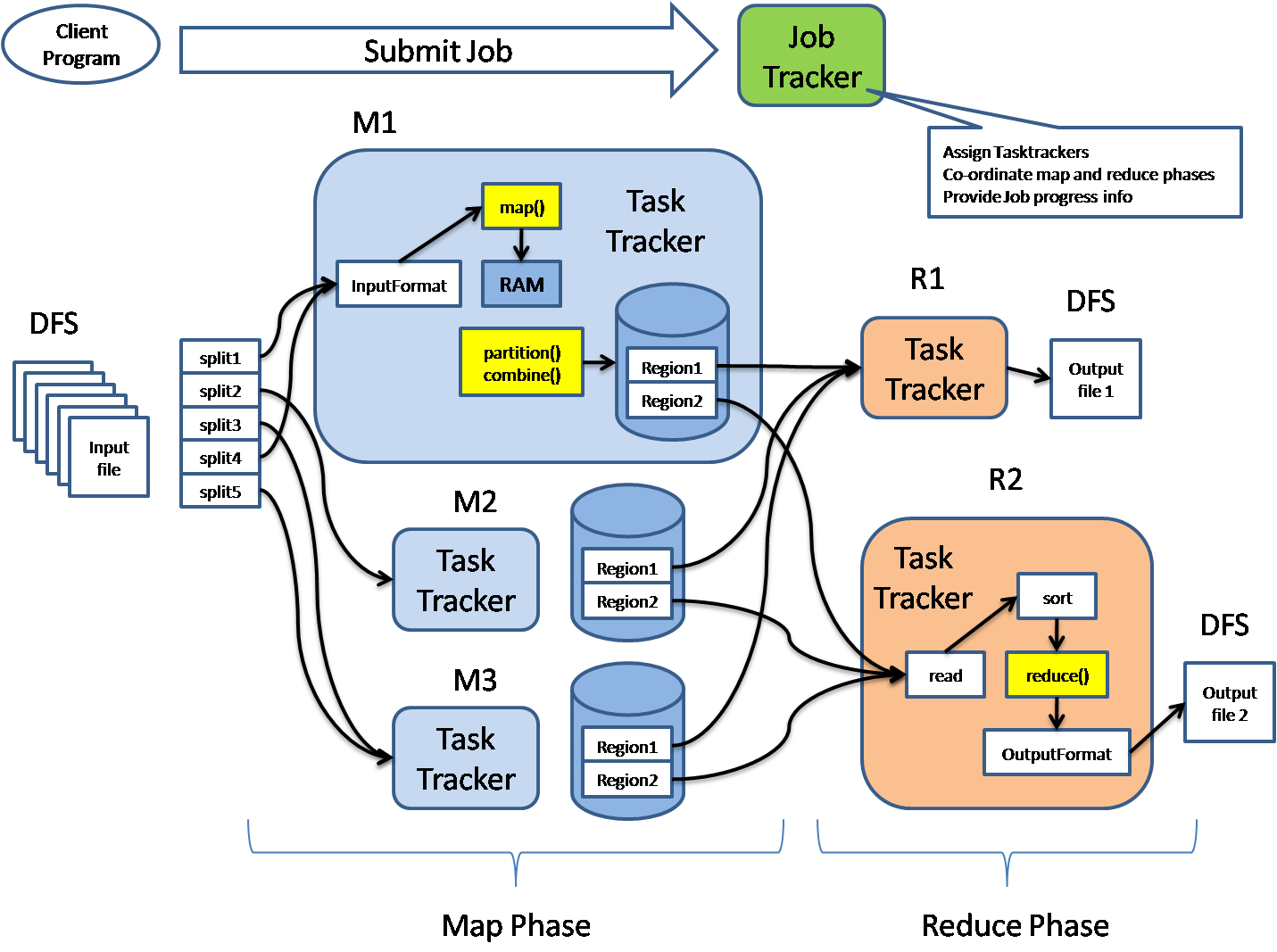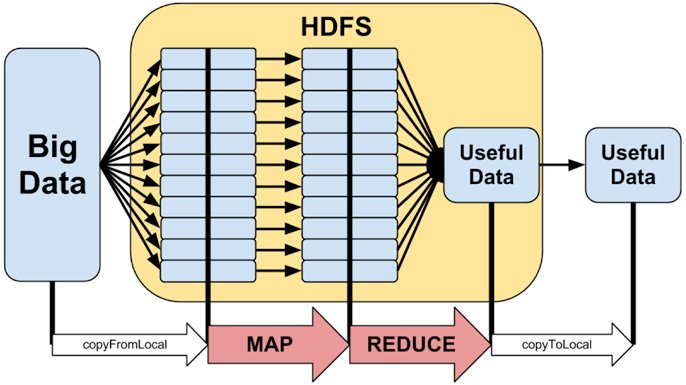# The world before Spark

## Parallelism

Parallelism is about speeding up computations by using multiple processors.

• Task parallelism: Different computations performed on the same data
• Data parallelism: Apply the same computation on dataset partitions

When processing big data, data parallelism is used to move computations close to the data, instead of moving data in the network.

## Issues with data parallelism

• Latency: Operations are 1.000x (disk) or 1.000.000x (network) slower than accessing data in memory
• (Partial) failures: Computations on 100s of machines may fail at any time

This means that our programming model and execution should hide (but not forget!) those.

## Map/Reduce

Map/Reduce is a general computation framework, loosely based on functional programming. It assumes that data exists in a K/V store

• `map((K, V), f: (K, V) -> (X, Y)): List[(X, Y)]`
• `reduce((K, List[V])): List[(X, Y)]`

Map/Reduce as a system was proposed by Dean & Ghemawat , along with the GFS.Hadoop was the first framework to enable computations to run on 1000s of commodity computers.

What is wrong: Performance

• Before each Map or Reduce step, Hadoop writes to HDFS
• Hard to express iterative problems in M/R
• All machine learning problems are iterative